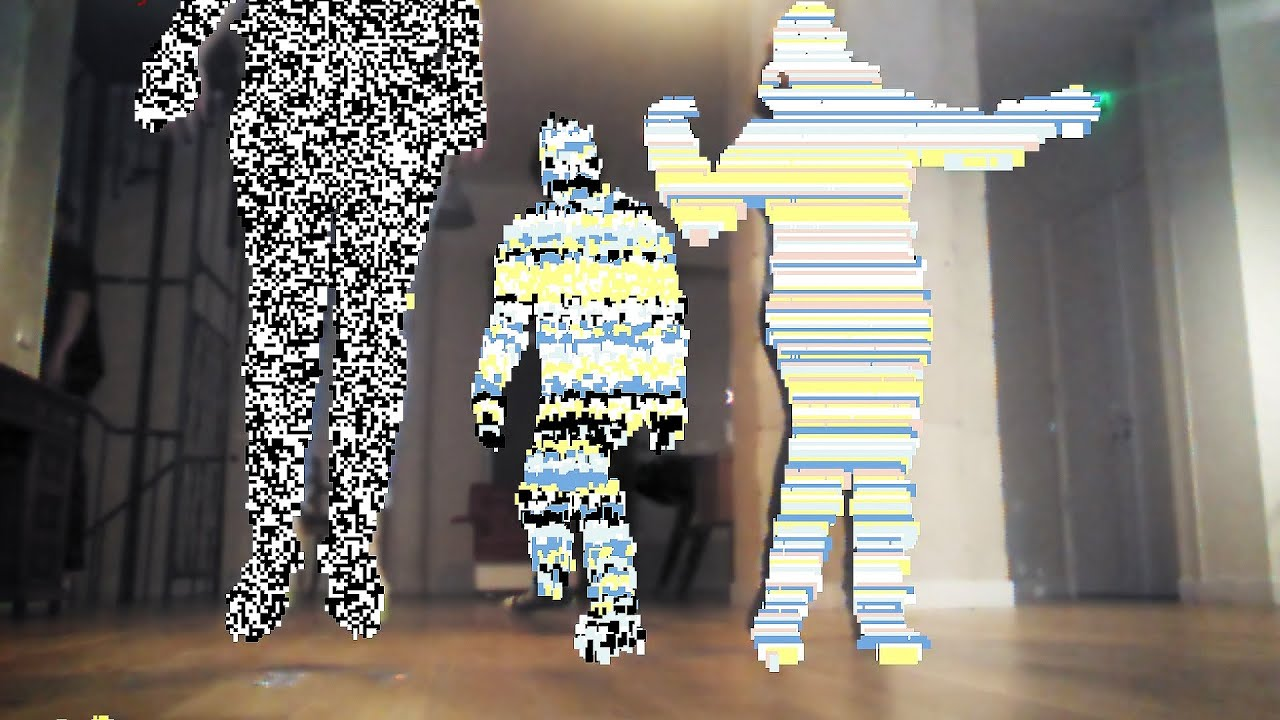#### Howdy, Stranger!

We are about to switch to a new forum software. Until then we have removed the registration on this forum.

# Get Usermap for each user

edited April 2018 in Kinect

Hi everyone,

I'm trying to make a processing program where each person (silhouette) will be assigned a different glitch. Essentially something like thisRight now we can only assign the same glitch for each person.

This is our code:

``````    class User
{
int userid;
int glitchid;

User(SimpleOpenNI curContext, int id)
{
glitchid = (int)random(1,2);
userid = id;
}

User()
{
}
}

import processing.opengl.*;
import SimpleOpenNI.*;

SimpleOpenNI  kinect;
int userID;
int[] userMap;
int randomNum;
float red;
float green;
float blue;
PImage rgbImage;

int count;
ArrayList <User> users = new ArrayList <User> ();

void setup() {
size(640, 480, P3D);
kinect = new SimpleOpenNI(this);
kinect.setMirror(true);
kinect.enableDepth();
kinect.enableUser();
kinect.enableRGB();
}

void draw() {
kinect.update();
rgbImage = kinect.rgbImage();
image(rgbImage, 0, 0);

int[] userList = kinect.getUsers();
for(int i=0;i<userList.length;i++)
{
int glitch =  users.get(i).glitchid;
if (glitch == 1)
{
crt();
}
else
{
crtcolor();
}
}

stroke(100);
smooth();
}

void onNewUser(SimpleOpenNI curContext, int userId)
{
println("onNewUser - userId: " + userId);
User u = new User(curContext, userId);
println("glitchid " + u.glitchid);
curContext.startTrackingSkeleton(userId);
}

void onLostUser(SimpleOpenNI curContext, int userId)
{
println("onLostUser - userId: " + userId);
}

void crtcolor()
{
color randomColor = color(random(255), random(255), random(255), 255);
boolean flag = false;
if (kinect.getNumberOfUsers() > 0) {
userMap = kinect.userMap();
for (int x = 0; x < width; x++) {
for (int y = 0; y < height; y++) {
int loc = x + y * width;
if (userMap[loc] !=0) {
if (random(100) < 50 || (flag == true && random(100) < 80))
{
flag = true;
color pixelColor = pixels[loc];
float mixPercentage = .5 + random(50)/100;
pixels[loc] =  lerpColor(pixelColor, randomColor, mixPercentage);
} else
{
flag = false;
randomColor = color(random(255), random(255), random(255), 255);
}
}
}
}
updatePixels();
}
}

void crt()
{
if (kinect.getNumberOfUsers() > 0) {
userMap = kinect.userMap();
for (int x = 0; x < width; x++) {
for (int y = 0; y < height; y++) {
int loc = x + y * width;
if (userMap[loc] !=0) {
pixels[loc] = color(random(255) );
}
}
}
updatePixels();
}
}
``````

I know that usermap will give me all the pixels and in my crt/crtcolor code i am changing all the pixels which is why it is not working. Is there a way/function that will give me the pixels associated with each user instead?

Tagged:

• Not an expert in kinect but I have few questions:

Do you detect when a new user enter or leaves the scene? Do you get your messages from `onNewUser()`/`onLostUser()`?

What documentation are you using for kinect? The question is to relate `kinect.userMap()` to each user as stored in `kinect.getUsers()`. I could suggest a solution by implementing your own userID assignation directly on your userMap pixel data (aka. detect your own users).... but no need to re-invent the wheel if it is already done for you by the library.

Also what kinect device are you using? I could help me if you provide the specs.

Kf

• We were able to figure it out, thank you for the help! The usermap can be indexed to let us know the userid and from there we can just mess around with the pixels

``````  rgbImage = kinect.rgbImage();
userMap = kinect.userMap();
for (int i = 0; i < userMap.length; i++)

{
if (userMap[i] == 1)
{
rgbImage.pixels[i]=color(random(255) );
}
else if (userMap[i] == 2)
{
rgbImage.pixels[i] = color(255,0,0);
}
}

rgbImage.updatePixels();
image(rgbImage, 0, 0);
``````